### Day 69 - Exponential Functions: Differentiation and Integration - 04.27.15

Update
• Unit 5 Test, this Friday!
• Go over previous Exit Ticket
1. Integration using the Natural Logarithmic Function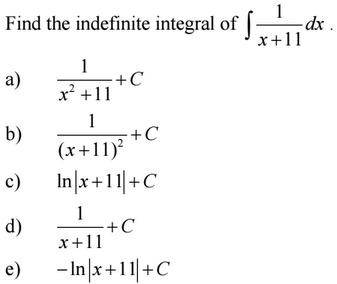1. Integration using the Natural Logarithmic Function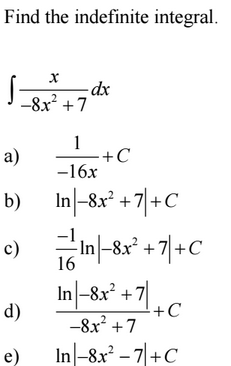1. Integration using the Natural Logarithmic Function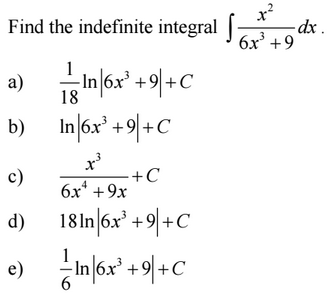1. Integration using the Natural Logarithmic Function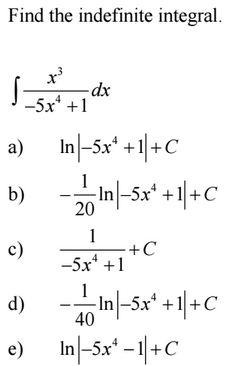1. Integration using the Natural Logarithmic Function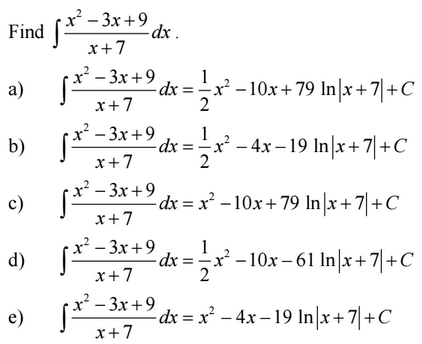Questions

Bell Ringer
1. Exponential Differentiation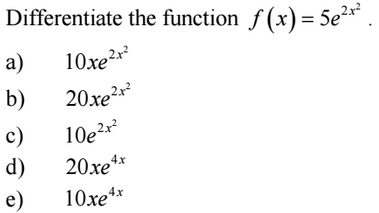1. Exponential Differentiation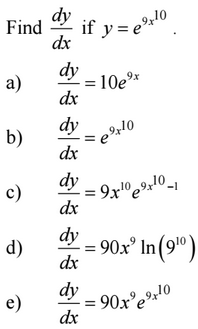1. Exponential Integration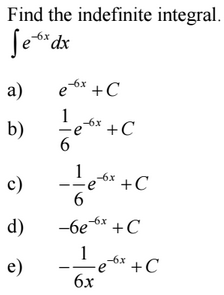1. Exponential Integration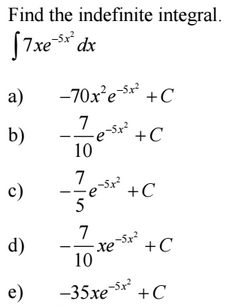1. Exponential Integration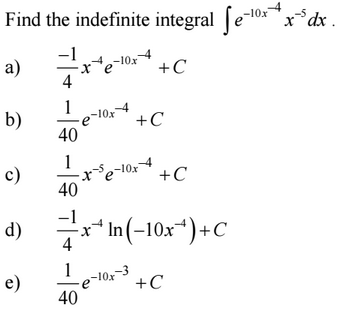Review
• Prerequisites
• Derivatives
• Derivative Rules
• Power Rule
• Constant Rule
• Trig Derivatives
• Logarithm Properties
• Differentiation using the Natural Logarithmic Functions (video)
• Integration using the Natural  Logarithmic Function (video)

Lesson
• Exponential Functions: Differentiation and Integration (video)

Exit Ticket
• Posted on the board at the end of the block!

Lesson Objective(s)
• How can the derivative of natural logarithmic functions be calculated?
Skills
1. Explain why the power rule does not work when integrating 1/x.
2. Calculate the derivative of the natural logarithmic function.

#### In-Class Help Requests

Standard(s)
• APC.9
• Apply formulas to find derivatives.
• Includes:
• derivatives of algebraic, trigonometric, exponential, logarithmic, and inverse trigonometric functions
• derivations of sums, products, quotients, inverses, and composites (chain rule) of elementary functions
• derivatives of implicitly defined functions
• higher order derivatives of algebraic, trigonometric, exponential, and logarithmic, functions